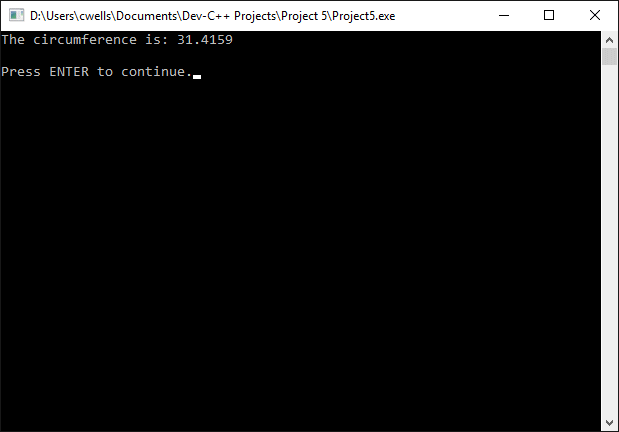Constants

Constants are expressions that have a fixed value. Unlike variables, their value cannot be changed by the program code once they have been declared. Constants can be numeric, character values or strings. Numeric values can be integer or floating point values, and can also be expressed as octal (base 8) or hexadecimal (base 16) numbers. Constants can be defined using the #define pre-processor directive, as follows:

#define PI 3.14159265

In the example above, PI is the identifier that will be used in the program to represent the constant value 3.14159265. The following short program demonstrates the use of this constant.

// Example Program - Constants

#include <iostream>
using namespace std;

#define PI 3.14159265

int main ()
{
double circ;     // circumference
string s;

circ = 2 * PI * rad;
cout << "The circumference is: ";
cout << circ;
cout << "\n\nPress ENTER to continue.";
getline( cin, s );
return 0;
}The output from the example program

The compiler replaces any occurrence of the identifier PI in the program with the floating point value 3.14159265. You can also use the const prefix to declare a constant with a specific data type, as shown below.

const double PI = 3.14159265;